• python round
千次阅读
2020-12-28 22:29:13

round( number ) 函数会返回浮点数 number 的四舍五入值。

具体定义为 round(number[,digits])：如果 digits>0 ，四舍五入到指定的小数位；

如果 digits=0 ，四舍五入到最接近的整数；

如果 digits<0 ，则在小数点左侧进行四舍五入；

如果 round() 函数只有 number 这个参数，则等同于 digits=0。

示例如下：

logging.info(round(9.315,2))

logging.info(round(9.3151,2))

logging.info(round(9.316,2))

logging.info(round(9.316,-1))

运行结果：

INFO - 9.31

INFO - 9.32

INFO - 9.32

INFO - 10.0注意： round(9.315,2)=9.31，并不是我们想的那样！只有 9.315 后面还有数字，才会进位，比如 round(9.3151,2)=9.32。

以上是 python3.x 的 round 函数说明。注意： python2.x 的 round 函数与 python 3.x 的 round 函数结果不同！

python2.x round 函数的官方定义为：Values are rounded to the closest multiple of 10 to the power minus ndigits; if two multiples are equally close, rounding is done away from 0. 即如果舍入处理的值，离左右两端相同距离，那么会远离 0，即为 1，所以 round(0.5)=1.0，而 round(-0.5)=-1。

python3.x round 函数的官方定义为：“values are rounded to the closest multiple of 10 to the power minus ndigits; if two multiples are equally close, rounding is done toward the even choice. 即如果舍入处理的值，离左右两端相同距离，那么会朝向偶数方向处理，所以 round(0.5)=1.0，而 round(-0.5)=1，也是 1！

更多相关内容
• python roundPython round() function is used to perform rounding operation on numbers. Python round（）函数用于对数字执行舍入运算。 python round（） (Python round()) Python round() function syntax is...

python round

Python round() function is used to perform rounding operation on numbers.

Python round（）函数用于对数字执行舍入运算。

## python round（） (Python round())

Python round() function syntax is:

Python round（）函数语法为：

round(number[, ndigits])

The number is rounded to ndigits precision after the decimal point.

数字在小数点后四舍五入为n位精度。

If ndigit is not provided or is None, then nearest integer is returned.

如果未提供ndigit或为None，则返回最接近的整数。

While rounding the input number to an integer, if both round up and round down values are equally close then even number is returned. For example, 10.5 will be rounded to 10 whereas 11.5 will be rounded to 12.

将输入数字四舍五入为整数时，如果四舍五入值和四舍五入值均相等，则返回偶数。 例如，将10.5舍入为10，而将11.5舍入为12。

Any integer value is valid for ndigits (positive, zero, or negative).

任何整数值都对n位数字有效（正数，零或负数）。

## Python round（）函数示例 (Python round() function examples)

Let’s look at some example of round() function.

让我们看一下round（）函数的一些示例。

round() to integer

round（）转换为整数

print(round(10, 2))

print(round(10.2))
print(round(10.8))
print(round(11.5))

Output:

输出：

10
10
11
12

round() to even side

将round（）整齐

# if both side of rounding is same, even is returned
print(round(10.5))
print(round(12.5))

Output:

输出：

10
12

round() with ndigit as None

round（），ndigit为无

print(round(1.5))
# OR
print(round(1.5, None))

Output:

输出：

2
2

round() with negative ndigit

负ndigit的round（）

print(round(100, 0))
print(round(100.1234, -4))
print(round(100.1234, -5))

Output:

输出：

100
100.0
0.0

## Python圆形浮点数 (Python round float)

When rounding is applied on floating point numbers, the result can be sometimes surprising. It’s because the numbers are stored in binary format and mostly decimal fractions cannot be represented exactly as binary fractions.

将四舍五入到浮点数上时 ，结果有时会令人惊讶。 这是因为数字以二进制格式存储，并且大多数十进制小数不能完全表示为二进制小数。

Python does the approximation and presents us the rounded value, because of this floating point arithmetic can sometimes result in surprising values.

Python会进行近似并为我们提供四舍五入的值，因为这种浮点算法有时会产生令人惊讶的值。

For example:

例如：

>>>.1 + .1 == .2
True
>>>.1 + .1 + .1 == .3
False
>>>.1 + .1 + .1 + .1 == .4
True

Let’s see some examples of round() function with floats.

让我们看一些带有float的round（）函数的示例。

print(round(2.675, 2))

print(round(1.2356, 2))
print(round(-1.2356, 2))

Output:

输出：

2.67
1.24
-1.24

Notice that first float rounding seems wrong. Ideally, it should be rounded to 2.68.

请注意，第一次浮点舍入似乎是错误的。 理想情况下，它应四舍五入为2.68。

This is the limitation of arithmetic operations with floats, we shouldn’t rely on conditional logic when dealing with floating point numbers.

这是浮点数算术运算的局限性，在处理浮点数时我们不应该依赖条件逻辑。

## round（）与自定义对象 (round() with custom object)

We can use round() function with a custom object too if they implement __round__() function. Let’s look at an example.

如果它们实现__round __（）函数，我们也可以将其与自定义对象一起使用。 让我们来看一个例子。

class Data:
id = 0

def __init__(self, i):
self.id = i

def __round__(self, n):
return round(self.id, n)

d = Data(10.5234)
print(round(d, 2))
print(round(d, 1))

Output:

输出：

10.52
10.5
GitHub Repository. GitHub存储库中检出完整的python脚本和更多Python示例。

Reference: Official Documentation

参考： 官方文档

python round

展开全文• 最近在刷NCT的PYTHON等级考试题目，在使用round这个非常不起眼的四舍五入常规函数时候发觉了一件很有意思的事情，即在某些时候.5并不会五入，反而变成了四舍。具体看下如下两个例子 在这两个例子我发觉很奇怪的...

首先来简单介绍下round这个函数。

1. 语法

round( x [, n]  )

2. 参数

• x -- 数值表达式。
• n -- 数值表达式，表示从小数点位数。

3. 返回值

返回浮点数x的四舍五入值。

4. 实例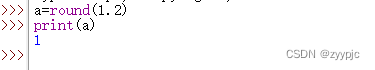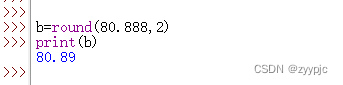最近在刷NCT的PYTHON等级考试题目，在使用round这个非常不起眼的四舍五入常规函数时候发觉了一件很有意思的事情，即在某些时候.5并不会五入，反而变成了四舍。具体看下如下两个例子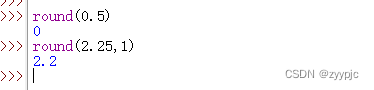在这两个例子我发觉很奇怪的现象，照道理0.5四舍五入应该是1，而2.25保留一位的四舍五入应该是2.3，而实际得到的结果与我们的数学尝试都不相符，这是为什么呢？？？

查阅了Python的文档后，发现这个问题其实是由Python版本所导致。这个问题只存在于Pyhon3中，而Python2中则一切正常。我们先来看下如下文档的描述。

在python2.7的doc中，round()的最后写着，"Values are rounded to the closest multiple of 10 to the power minus ndigits; if two multiples are equally close, rounding is done away from 0." 保留值将保留到离上一位更近的一端（四舍六入），如果距离两端一样远，则保留到离0远的一边。所以round(0.5)会近似到1，而round(-0.5)会近似到-1。

但是到了python3.5的doc中，文档变成了"values are rounded to the closest multiple of 10 to the power minus ndigits; if two multiples are equally close, rounding is done toward the even choice." 如果距离两边一样远，会保留到偶数的一边。比如round(0.5)和round(-0.5)都会保留到0，而round(1.5)会保留到2。

紧接着我们分别在Python2/3中做实验去验证一下

版本:Python 3.10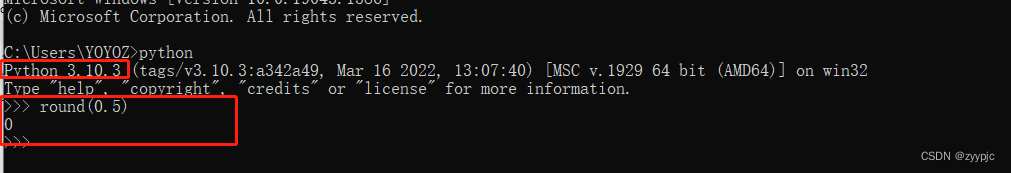版本:Python 2.7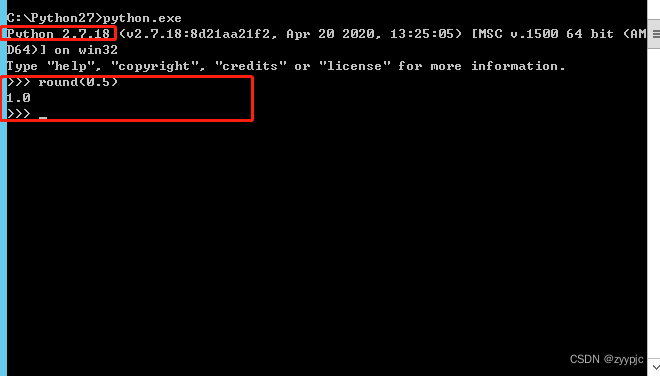# 所以如果有项目是从python2.x迁移到python3.x的，可要注意一下round的地方（当然，还要注意/和//，还有print，还有一些比较另类的库）！！！！！！

### 基于目前存在的这种情况，尽量避免使用round函数，我通过网络整理了下如下可供大家参考

• 使用math模块中的一些函数，比如math.ceiling（天花板除法）。
• python自带整除，python2中是/，3中是//，还有div函数。
• 字符串格式化可以做截断使用，例如 "%.2f" % value（保留两位小数并变成字符串……如果还想用浮点数请披上float()的外衣）。
• 当然，对浮点数精度要求如果很高的话，请用嘚瑟馍，不对不对，请用decimal模块。
展开全文python
• 关于round函数的详细用法和一些由于机器数问题产生的错误情况 round(number[, ndigits]) 返回number舍入到小数点后ndigits为精度的值，如果ndigits被省略则会返回最接近输入值的整数

round(number[, ndigits])
返回number舍入到小数点后ndigits为精度的值，如果ndigits被省略则会返回最接近输入值的整数

强调：round()的舍入规则不是四舍五入
强调：round()的舍入规则不是四舍五入
强调：round()的舍入规则不是四舍五入

舍入的规则
官方定义：结果值会舍入至最接近的 10 的负 ndigits 次幂的倍数；如果与两个倍数同样接近，则选用偶数。
有点不好理解，换一种理解方式
结果值 = 与number 最接近的拥有ndigits位小数的小数 。如果距离相等，则选择小数点第ndigits位是偶数的小数

• 如果不填ndigits 则保留整数部分 当出现距离相等的情况，则看整数末尾，最后结果靠近整数末尾是偶数的
round(number)
number在[1.5，2.5] 的值结果为2 1.5距离1和距离2相等，2是偶数所以结果是2，2.5距离2和距离3相等，2是偶数所以结果是2
number在 (2.5,3.5) 的值结果为3 在这个范围中的距离3最近所以结果为3
number=3.5 保留结果为4（3.5距离3和距离4都为0.5 因为4是偶数，所以最后的结果是4）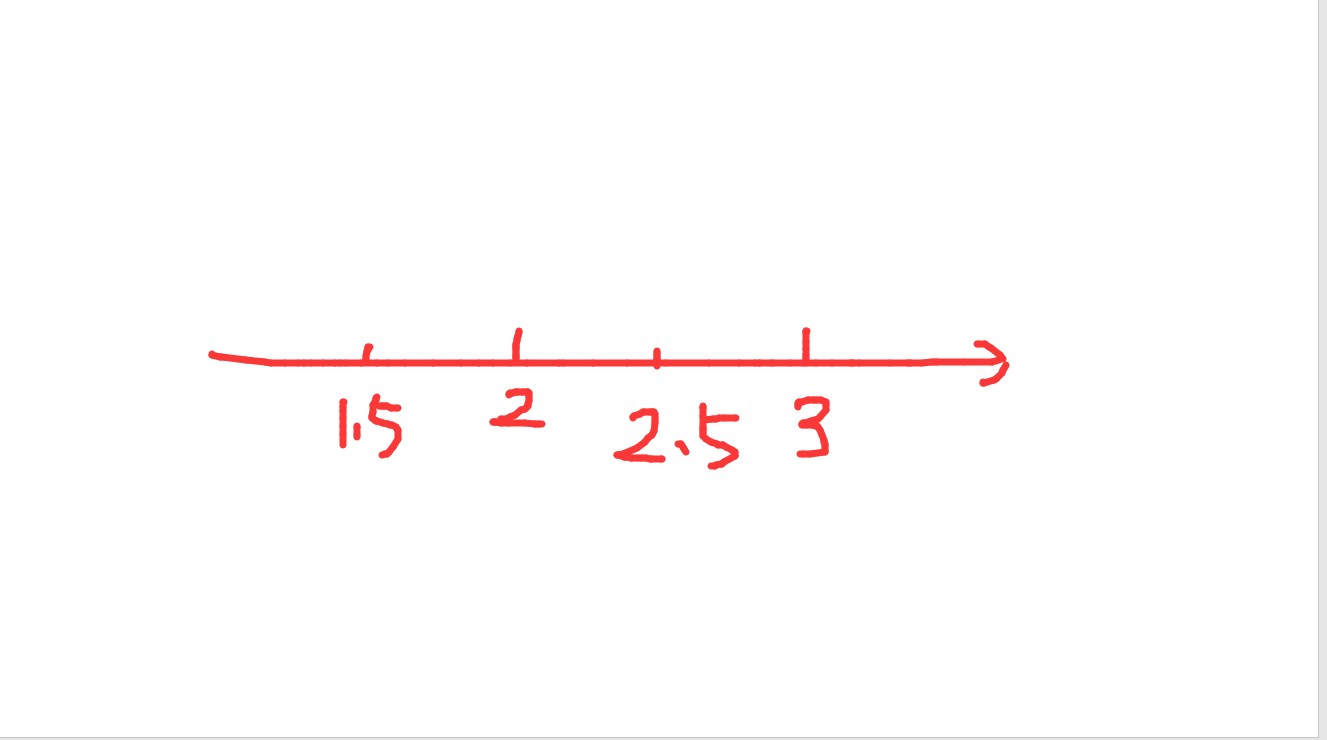特别注意：round(0.5) 和 round(-0.5) 均得出 0
• 如果有指定的ndigit值，则会保留对应的小数位方法和整数的相同
• 结果值等于和原值小数最接近的拥有ndigits位小数的值，如果距离相等选择小数点第ndigits位是偶数的值
a = 2.465 # 舍入后 2.46     # 距离2.46和2.47一样近，2.46的末尾小数为偶数，则结果为2.46
b = 2.4651  # 舍入后 2.47   # 距离2.47较近
print(round(a,2))
print(round(b,2))

a = 2.464 # 舍入后 2.46   # 距离2.46最近
b = 2.467  # 舍入后 2.47  # 距离2，47最近
print(round(a,2))
print(round(b,2))



### round()函数的坑

官方文档的例子

对浮点数执行 round() 的行为可能会令人惊讶：例如，round(2.675, 2) 将给出 2.67 而不是期望的 2.68。
这不算是程序错误：这一结果是由于大多数十进制小数实际上都不能以浮点数精确地表示。

因为所有的数据在计算机中都以二进制的形式进行存储，从十进制转化为二进制的过成中，会有精度的损失。
2.675转换成二进制以后在计算机存储的机器数要小于2.675 所以会导致结果和预期值不一样

如果想要精确度比较高的

• 使用模块 decimal
decimal可以调整小数点位数的进度

• format函数转换成字符串，可以指定保留小数位数，保留规则为四舍五入

展开全文python
• 分享了Python中的精确小数计算。decimal模块及round函数的四舍五入经常遇见的问题。python decimal
• 今天在Pycharm编译器中使用round()函数的时候编译器提示了警告，后经修改去掉了警告，这里做一下记录。 代码如下： import numpy as np x = np.array([0, 1]) y = np.sum(np.sin(x)) print(f"x四捨六入五留双后的值...
• Python中如何对float和double等多精度参数保留小数位，其中，细细数来一定有很多方法，我个人认为，方法贵精不贵多，逮着一个方法用就行，反而还记得住。 我用的最多的就是round(a,b)函数，其中a是输入的数值，b就是...python
• Python round()函数描述round() 方法返回浮点数x的四舍五入值。语法以下是 round() 方法的语法:参数x -- 数值表达式。返回值返回浮python
• Python提供了一个内置函数round()，该函数会四舍五入为给定的位数，并返回浮点数，如果没有提供四舍五入的位数，则会将数字四舍五入为最接近的整数。用法:round(number, number of digits)round()参数：..1) number ...
• print(round(2.5))#2 print(round(3.5))#4 print(round(-2.5))#-2 print(round(-3.5))#-4 这是因为从统计学的角度上来讲,如果大量数据采用四舍五入会造成统计结果偏大。而"奇进偶舍"可以将舍入误差降到最低。 举例 ...
• round( )函数简介 菜鸟教程中介绍到，round() 函数作用就是，返回浮点数x的四舍五入值。 > round( x [, n] ) 参数x，n均为数值表达式，返回值为x的四舍五入值。n为保留的小数位数，不加n则只保留x四舍五入后的整数...
• 解决Python round函数，奇进偶不进的问题 def newRound(data,num): import math try: data = float(data) except : return "非数字不能处理" return int((data * math.pow(10,int(num)) + 0.5))/math.pow(10,...python
• Python round(x,y) print(round(80.23456, 4)) # 将x进行四舍五入，在小数点后第y位 80.2346python
• 描述 round() 方法返回浮点数x的四舍五入值。 语法 以下是 round() 方法的语法: round( x [, n] ) .../usr/bin/python print "round(80.23456, 2) : ", round(80.23456, 2) print "round(100.000056计算机视觉 机器学习
• 强烈建议参考：为什么你需要少看垃圾博客以及如何在Python里精确地四舍五入
• 研究主题: Python中的round()函数用法及结果 Python版本信息: Python 3.10.2 (main, Feb 2 2022, 06:19:27) [Clang 13.0.0 (clang-1300.0.29.3)] IPython 8.2.0 – An enhanced Interactive Python. Type ‘?’ for...python
• ## pythonround函数

万次阅读 多人点赞 2019-01-24 13:00:59
round(number,num_digits) Number 需要进行四舍五入的数字。 Num_digits 指定的位数，按此位数进行四舍五入。 注解 如果 num_digits 大于 0，则四舍五入到指定的小数位。 如果 num_digits 等于 0，则四舍五入到最...
•python
• ## Python中round函数

千次阅读 2021-11-02 11:51:59
Pythonround函数Python round()描述语法参数实例补充round函数并不总是四舍五入参数n Python round() 描述 返回浮点数 x 的四舍五入值 语法 round(x[, n]) 参数 x：数值表达式 n：数值表达式，表示精确到...python
• Python 3.6.1 (default, Sep 7 2017, 16:36:03) [GCC 6.3.0 20170406] on linux Type "help", "copyright", "credits" or "license" for more information. >>> round(3.5) 4 >>> round(3.55, .....
• 感谢邱工提供的文档--------------------- 作者：qxq-xq 来源：CSDN ...版权声明：本文为博主原创文章，转载请附上博文链接！...Python round() 函数 round() 方法返回浮点数x的四舍五入值。 语法 round( x [,...
• ## 详解python中的round()函数

万次阅读 多人点赞 2022-03-02 18:27:11
round()是python自带的一个函数，用于数字的四舍五入。 使用方法（环境python3） round(number,digits) 参数： digits>0，四舍五入到指定的小数位 digits=0, 四舍五入到最接近的整数 digits<0 ，在小数点左侧...python
• https://www.runoob.com/python/func-number-round.html
• 最近在Python中使用round()函数取整然后后处理乘以100时，发现了一个奇怪的问题，如下： >>> round(61 / 154 * 100, 2) 39.61 >>> >>> (round(61 / 154, 2) )* 100 40.0 >>> ...python round函数
• python3中，round(1.0/2.0)得到的是1，而在python2中，round(1.0/2.0)得到的是0。例如$pythonPython 2.7.8 (default, Jun 18 2015, 18:54:19)[GCC 4.9.1] on linux2Type "help", "copyright", "credits" or "lic.... • python3中，round(1.0/2.0)得到的是1，而在python2中，round(1.0/2.0)得到的是0$pythonPython2.7.8(default,Jun182015,18:54:19)[GCC4.9.1]onlinux2Type"help","copyright","credits"or"licens...
• 使用round函数的时候发现了一个奇怪的现象。一直觉得round函数是一个用于四舍五入的函数，结果却不一定。一般如果觉得奇怪，那就是没弄懂其...在Python3中，round(1.0/2.0)得到的是1，而在Python2中，round(1.0/2...
• 摘要：在本文中，介绍了什么是round函数以及如何从python内核中实现它。同时，还介绍舍入函数的一些缺点，以及如何纠正它们，如何在数据科学中广泛使用的库中发挥作用。python 舍入函数
• round函数很简单，对浮点数进行近似取值，保留几位小数。比如>>> round(10.0/3, 2)3.33>>>...1、round的结果跟python版本有关我们来看看python2和python3中有什么不同：\$ pyt...
• ## pythonround函数用法

万次阅读 2016-11-25 13:48:27
round()方法返回 x 的小数点四舍五入到n个数字。语法 以下是round()方法的语法：round( x [, n] ) 参数 x –这是一个数值表达式n –这也是一个数值表达式返回值 该方法返回 x 的小数点四舍五入到n个数字例子 ...python...